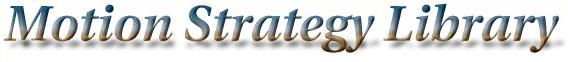Main Page   Namespace List   Class Hierarchy   Compound List   File List   Compound Members   File Members

# MSLEdge Class Reference

`#include <graph.h>`

List of all members.

## Public Methods

MSLEdge ()
MSLEdge (MSLVertex *v1, MSLVertex *v2, const MSLVector &u, double t)
MSLEdge (MSLVertex *v1, MSLVertex *v2, double t)
MSLEdge (MSLVertex *v1, MSLVertex *v2)
~MSLEdge ()
double Time () const
The time required to reach this node from the parent. More...

double Cost () const
A cost value, useful in some algorithms. More...

void SetCost (const double &x)
A cost value, useful in some algorithms. More...

MSLVector Input () const
An input vector that leads from the first state to the second. More...

MSLVertexSource () const
The time required to reach this node from the parent. More...

MSLVertexTarget () const
The time required to reach this node from the parent. More...

## Friends

istream & operator>> (istream &is, MSLEdge &e)
ostream & operator<< (ostream &os, const MSLEdge &e)

## Constructor & Destructor Documentation

 MSLEdge::MSLEdge ( )

 MSLEdge::MSLEdge ( MSLVertex * v1, MSLVertex * v2, const MSLVector & u, double t )

 MSLEdge::MSLEdge ( MSLVertex * v1, MSLVertex * v2, double t )

 MSLEdge::MSLEdge ( MSLVertex * v1, MSLVertex * v2 )

 MSLEdge::~MSLEdge ( ) ` [inline]`

## Member Function Documentation

 double MSLEdge::Cost ( ) const` [inline]`
 A cost value, useful in some algorithms.

 MSLVector MSLEdge::Input ( ) const` [inline]`
 An input vector that leads from the first state to the second.

 void MSLEdge::SetCost ( const double & x ) ` [inline]`
 A cost value, useful in some algorithms.

 MSLVertex* MSLEdge::Source ( ) const` [inline]`
 The time required to reach this node from the parent.

 MSLVertex* MSLEdge::Target ( ) const` [inline]`
 The time required to reach this node from the parent.

 double MSLEdge::Time ( ) const` [inline]`
 The time required to reach this node from the parent.

## Friends And Related Function Documentation

 ostream& operator<< ( ostream & os, const MSLEdge & e ) ` [friend]`

 istream& operator>> ( istream & is, MSLEdge & e ) ` [friend]`

The documentation for this class was generated from the following files: Motion Strategy Library

Web page maintained by Steve LaValle